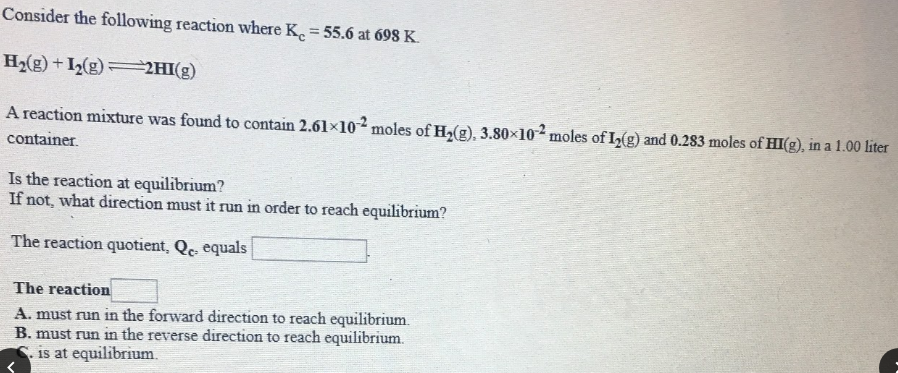# Consider the following reaction where Kc = 55.6 at 698 K. H2(g) + I2(g) ⇌ 2HI(g) A reaction mixture was found to contain 2.61x10^-2 moles of H2(g), 3.80x10^-2 moles of I2(g), and 0.283 moles of HI2(g), in a 1.00 liter container. Is the reaction at equilibrium? If not, what direction must it run in order to reach equilibrium? The reaction quotient, Qc, equals ____. The reaction ____.